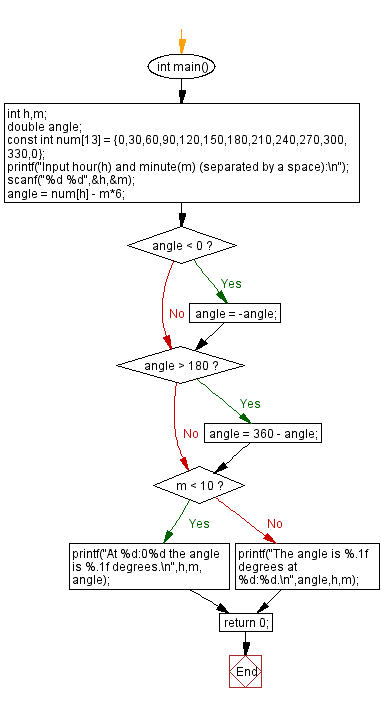﻿ C : Angle between the hour and the minute hand of a clock

# C Exercises: Find the angle between (12:00 to 11:59) the hour hand and the minute hand of a clock

## C Basic Declarations and Expressions: Exercise-91 with Solution

Write a C program to find the angle between (12:00 to 11:59) the hour hand and the minute hand of a clock. The hour hand and the minute hand are always between 0 and 180 degrees.
For example, when it's 12 o'clock, the angle of the two hands is 0 while 3:00 is 45 degrees and 6:00 is 180 degrees.

Sample Solution:

C Code:

``````#include <stdio.h>
#include <math.h>
#include <stdlib.h>

int main()
{
int h, m; // Declare variables for hour and minute
double angle; // Declare variable for angle

const int num = {0,30,60,90,120,150,180,210,240,270,300,330,0}; // Define an array of angles

printf("Input hour(h) and minute(m) (separated by a space):\n"); // Prompt user for input
scanf("%d %d",&h,&m); // Read input for hour and minute

angle = num[h] - m*5.5; // Calculate the angle based on the input values

if (angle < 0) // Ensure angle is positive
angle = -angle;

if (angle > 180) // Ensure angle is within 180 degrees
angle = 360 - angle;

if ( m < 10 )
printf("At %d:0%d the angle is %.1f degrees.\n",h,m,angle); // Print angle and time in format HH:MM
else
printf("The angle is %.1f degrees at %d:%d.\n",angle,h,m); // Print angle and time in format HH:MM

return 0; // Indicate successful execution of the program
}
``````

Sample Output:

```Input hour(h) and minute(m) (separated by a space):
3 0
At 3:00 the angle is 90.0 degrees.
Input hour(h) and minute(m) (separated by a space):
6 15
The angle is 90.0 degrees at 6:15.
Input hour(h) and minute(m) (separated by a space):
12 0
At 12:00 the angle is 0.0 degrees.
```

Flowchart:C programming Code Editor:

What is the difficulty level of this exercise?

Test your Programming skills with w3resource's quiz.

﻿